# Fractions + rational numbers - math problems

#### Number of problems found: 73

• Simplify 9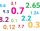Simplify and express the result as a rational number in its simplest form 1/2+ 1/5+ 6.25+0.25
• Write 4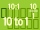Write each ratio as a fraction of whole numbers: a 4.8 to 11.2 6) 2.7 to 0.54
• The expressionWhat is the value of the expression ((62+60))/(23)
• Two fraction equation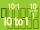Find d value of q in the equation 30/16=q/48.
• Simplest form of a fractionWhich one of the following fraction after reducing in simplest form is not equal to 3/2? a) 15/20 b) 12/8 c) 27/18 d) 6/4
• Two numbers 11The sum of two rational numbers is (-2). If one of them is 3/5, find the other.
• The length 6The length of 12 pipes is 10 1/2 meters. (1) find the length of one pipe (2) also find the length of 7 pipes
• Bubble bee marblesMark has 100 marbles. Seventeen are bubble bee marbles. What decimal number shows the fraction of marbles that are bumblebees?
• Distributive propertyVerify the distributive property a×(b+c)=(a×b)+(a*c) for the rational number a=5/8, b=7/4 and c=2/3
• Boys and girlsIf the ratio of male & female teachers is 2/5 & it is in proportion of the male & female students, how many are girls if there are 42 boys?
• Two numbers and its productThe product of two numbers are 2/3. If on of them is 1/10, what is the other?
• Tom has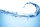Tom has a water tank that holds 5 gallons of water. Tom uses water from a full tank to fill 6 bottles that each hold 16 ounces and a pitcher that holds 1/2 gallon. How many ounces of water are left in the water tank?
• ArrangeArrange the following in descending order: 0.32, 2on5, 27%, 1 on 3
• Between two mixed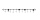What is the rational number between 2 1/4 and 2 4/5?
• Submerging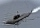Monika dove 9 meters below the ocean's surface. She then dove 13 meters deeper. Then she rose 19 and one-fourth meters. What was her position concerning the water's surface (the water surface = 0, minus values = above water level, plus = above water level
• The elevation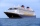The elevation of a sunken ship is -120 feet. Your elevation is 5/8 of the ship’s elevation. What is your elevation?
• Flowers 4A flower seller has 4.05 kg of orange flowers and 6.50 kg of red flowers. He made flower baskets with 2.11 kg of both red and orange flowers in each. How many baskets did he make?
• ThousandthsIf you have 0.08 what is the form in thousandths?
• Colored blocksTucker and his classmates placed colored blocks on a scale during a science lab. The brown block weighed 8.94 pounds, and the red block weighed 1.87 pounds. How much more did the brown block weigh than the red block?
• What is 11What is the quotient of Three-fifths and 1 Over 10?

Do you have an exciting math question or word problem that you can't solve? Ask a question or post a math problem, and we can try to solve it.

We will send a solution to your e-mail address. Solved examples are also published here. Please enter the e-mail correctly and check whether you don't have a full mailbox.

Need help to calculate sum, simplify or multiply fractions? Try our fraction calculator. Fraction Word Problems. Rational numbers - math problems.